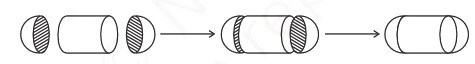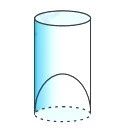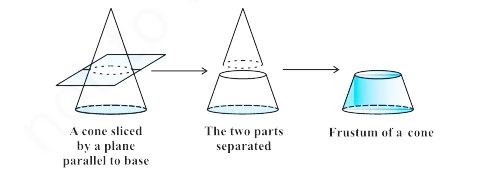or# Surface Areas And Volumes NCERT Solutions : Class 10 Maths

## NCERT Solutions for Class 10 Maths : Surface Areas And VolumesFilter
Filters :
Classes
•
•
•
•
•
•
•
Chapters
•
•
•
•
•
•
•
•
•
•
• 4 More
Exercises
•
•
•
•
•
•

### NCERT Class 10 | SURFACE AREAS AND VOLUMES | Exercise 04 | Question No. 03

A fez, the cap used by the Turks, is shaped like the frustum of a cone. If its radius on the open side is 10 cm, radius at the upper base is 4 cm and its slant height is 15 cm, find the area of material used for making it.### NCERT Class 10 | SURFACE AREAS AND VOLUMES | Exercise 04 | Question No. 05

A metallic right circular cone 20 cm high and whose vertical angle is `60o` is cut into two parts at the middle of its height by a plane parallel to its base. If the frustum so obtained be drawn into a wire of diameter `1/(16)c m` find the le### NCERT Class 10 | SURFACE AREAS AND VOLUMES | Exercise 04 | Question No. 04

A container, opened from the top and made up of a metal sheet, is in the form of a frustum of a cone of height 16 cm with radii of its lower and upper ends as 8 cm and 20 cm, respectively. Find the cost of the milk which can completely fill the cDoubtnut one of the best online education platform provides free NCERT Solutions of Maths for Class 10 Surface Areas And Volumes which are solved by our maths experts as per the NCERT (CBSE) guidelines. We provide the solutions as video solutions in which concepts are also explained along with answer which will help students while learning , preparing for exams and in doing homework. These Solutions will help to revise complete syllabus and score more marks in examinations.

Get here free, accurate and comprehensive NCERT Solutions for Class 10 Maths Surface Areas And Volumes which have been reviewed by our maths counsellors as per the latest edition following up the CBSE guidelines. We provide video solutions in which solutions to all the questions of NCERT Class 10 Maths textbook are explained in step by step and detailed way.

## The Topics of the Chapter Surface Areas And Volumes are :

EXAMPLES OF FRUSTUM

SURFACE AREAS AND VOLUMES OF COMBINATIONS OF SOLIDS

FRUSTUM OF RIGHT CIRCULAR CONE

CONVERSION OF SOLIDS

INTRODUCTION

It contains these exercises along with solved examples. All the exercises are solved in the video. Select the exercise to view the solutions exercisewise of the Chapter Surface Areas And Volumes:

## NCERT Solutions Class 10 Maths Chapter Surface Areas And Volumes Exercises:

We have covered all the exercises and also Solved examples in the videos. Along with the practise exercise Students should also practise solved examples to clear the concepts of the Surface Areas And Volumes. If incase you have any doubt you can watch the solutions for the given questions.Watch the solutions for the given questions in which it is also explained in the video steps to solve the questions along with answers.

In this chapter, we will determine the surface area and volume of an object formed by joining any two of the basic solids, like cuboid, cylinder, cone, sphere and hemisphere. Getting all the right idea on how to work on it in the perfect manner is made much easier by the team of Doubtnut. The reason behind this is the fact that students get to work on the different exercises where they can also follow lots of examples as well. So, it proves to be quite helpful in getting all the perfect idea on how to work on the different problems without any worry at all.

During exams, students find it quite difficult in attempting certain tricky questions. The reason behind this is the fact that NCERT textbooks that are prescribed for the students are not able to provide clear and in-depth concepts that lead to a lot of problem in scoring good marks in the exam.  Therefore, the only options that are left for them is to look forward to the different reference books or conventional tutors. It leads the students to shell out a hefty amount of money and time. Therefore, Doubtnut provides the video solutions at free of cost and the video solutions are structured for easy and quick learning.

Students can really make the optimum use of the study material prepared by the experts of Doubtnut. The video tutorials enable the students to solve different questions without taking much time. So, with the right and proper concept with the help of the experts, it becomes quite easy to get the precise and swift solutions. The experts have made use of step by step instructions which proves to be an added advantage in learning process. The NCERT solutions are provided in the pdf format as well. Students can download PDFs and practice math questions even without internet. So, students who are really serious in achieving success in the exams should start learning math from solutions and study material provided by doubtnut. Moreover, Class 10 results play a vital role in getting admission in higher secondary schools and getting subject stream of your preference. CBSE Board exam results when mentioned on the student’s resume indicate academic performance and achievements.

## Topics and subtopics included in NCERT Solutions for Class 10 Maths Chapter 13 Surface areas and volumes

### Introduction

You are already familiar with the solid shapes cone, cylinder, cuboid, sphere, etc. It has also been possible to know how to find their surface areas and volumes. In our daily life, we see a number of solids that are made up of two or more of the basic solids. You might have seen a test tube, right? Well, you should know that the tube happens to be a combination of a cylinder and a hemisphere. So, in order to find the surface areas or capacities or volumes of such objects, you need to know how to do it in the best way. So, in this chapter, you would be able to get the right idea as to how you can determine the volume and surface area of such object without facing any difficulty.

### Surface Area of a Combination of Solids

In order to find the clear idea about the surface area of a solid like the below figure, we should try to break it down into different parts.Here, we can see that the solid is made up of a cylinder where you can find there are two hemispheres which are stuck at either end. So, you can find that the above figure will appear if you happen to put the pieces all together.

Thus, you can say that the sum of the curved surface areas of each of the individual parts happens to be the total surface area of the new solid.

Therefore, Total Surface Area = CSA of one hemisphere +CSA of cylinder + CSA of other hemisphere where CSA refers to Curved Surface Area.

### Volume of a Combination of Solids

Here, we will get a good idea about how to calculate the volume of a combination of solids. Let’s take an example:

A juice seller was serving his customers using glasses as shown in the below figure:The inner diameter of the cylindrical glass was 5 cm, but the bottom of the glass had a hemispherical raised portion which reduced the capacity of the glass. If the height of a glass was 10 cm, find the apparent capacity of the glass and its actual capacity. (Use π = 3.14.)

Solution: You can see that the inner diameter of the glass = 5 cm and height = 10 cm, the apparent capacity of the glass = πr2h = 3.14 × 2.5 × 2.5 × 10 cm3 = 196.25 cm3

But at the same time, when it comes to the actual capacity of the glass, it is found to be less by the volume of the hemisphere at the base.

So, it proves to be less by 2 πr3 = 2 x 3.14 x 2.5 x 2.5 x 2.5 cm3 =32.71cm3

3         3

Thus it can be said that the actual capacity of the glass = apparent capacity of glass – volume of the hemisphere

= (196.25 – 32.71) cm3

= 163.54 cm3

Conversion of Solid from One Shape to Another

You have seen candles which are generally in the shape of a cylinder. It is also possible to find candles that arrive in the shapes of animals. Do you have any idea as to how it is made? Well, in this case, the wax needs to be heated in a metal container until it turns into the liquid form. The next thing that you need to do is to get a container that has an animal or any other shape and pour into it. So, after it cools down, it takes the shape of the particular animal or any other object.

Let’s take an example:

With the help of modeling clay, a cone is made with height as 24cm and radius of base 6cm. How can you find the radius of the sphere?

Solution:   As we know that:

Volume of cone = 1 x π x 6 x 6 x 24cm3

3

By taking r as the radius of the sphere, then volume is 4 πr3

3

For both in the form of cone and the sphere, the volume of clay remains the same, we have,

4 x π x r3 = 1 x π x 6 x 6 x 24

3

i.e.                              r3 = 3 x 3 x 24 =33 x 23

So,                              r = 3 x 2 = 6

So, the radius of the sphere is 6cm.

### Frustum of a Cone

Here, we will try to take a right circular cone and try to remove a portion of it. It is found that there are many ways to do it. Well, have a look at this:

Take any plasticine material or some clay and form a cone. Now you need to cut it with a knife and you should do it to make sure that it has been cut parallel to its base. Now you need to remove the smaller cone. Now find out as to what you are left with. You would be able to find a solid which is known as the frustum of the cone. It can be seen that there are two circular ends and that too with different radii.## Significance of Doubtnut for Class 10 students

The video solutions and PDFs provided by Doubtnut help the students to save both money and time. It also provides the most useful Class 10 Maths NCERT Solutions that proves to be of quite help to them. So, it really helps to achieve success in the exams in the best way. Let us have a look at the different benefits that students get to enjoy:

• Boosts self-confidence: Students can find that by practicing the different questions, it has helped them in getting the accurate idea on how to come up with the accurate solutions. So, this makes it possible to boost their level of confidence that truly makes it possible to achieve success in the exams without any worry at all.
• Ease of learning: It is possible for the students to download the online study materials in the form of pdf. So, this helps a lot to study according to their convenience. It also does not matter at all as to whether they are traveling or at any other place. Therefore, it becomes possible to get all the perfect information on how to attempt the different questions without any worry at all.
• Provides the ultimate support: Getting hold of the perfect support is also possible by the team of Doubtnut which helps a lot to stay away from any sort of confusions. If students come across any doubts while learning math, all they have to do is post a picture of doubt question from the textbook on our platform and the students will receive solution in seconds.